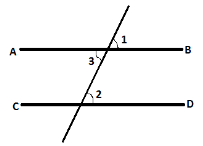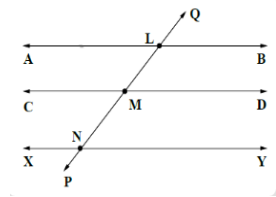QuestionAnswers

# State whether the given statement is true or false. Two lines which are parallel to a common line are parallel to each other.Verified
128.4k+ views
Hint: Here in this question some properties of parallel lines will get used which are being mentioned below with the help of a diagram.Alternate angles. ($\angle 3 = \angle 2$ )
Corresponding angles. ($\angle 1 = \angle 2$ )
Vertical opposite angles. ($\angle 1 = \angle 3$)Suppose three lines are there naming AB, CD and XY. Two lines AB and XY are parallel to CD. So we have to prove that they are parallel to each other also. For this we will draw a transversal line PQ such that it cuts AB at point L, CD at point M and XY at point N.
$AB\parallel CD$ and PQ is a transversal line.
$\therefore \angle BLM = \angle DMP$ (Corresponding angles) ....................equation 1.
$XY\parallel CD$ and PQ is a transversal line.
$\therefore \angle YNP = \angle DMP$ (Corresponding angles) ...................equation 2.
From equation 1 and 2 we get that $\angle BLM = \angle YNP$
Therefore from the reverse statement of property of parallel lines which states that if two lines are parallel then corresponding angles are equal similarly when corresponding angles are equal then the lines through which angles are there will be parallel.
$AB$ is parallel to $XY$
The given statement is true.

Note: Students may find little confusion in determining corresponding and alternate angles so in hint basic diagram which is mentioned must be remembered.
Alternate method for this question: -
$AB\parallel CD$ and PQ is a transversal line.
$\therefore \angle ALM = \angle CMN$ (Corresponding angles) ....................equation 1.
$XY\parallel CD$and PQ is a transversal line.
$\therefore \angle XNP = \angle CMN$ (Corresponding angles) ...................equation 2.
From equation 1 and 2 we get that $\angle ALM = \angle XNP$
Therefore from the reverse statement of property of parallel lines which states that if two lines are parallel then corresponding angles are equal similarly when corresponding angles are equal then the lines through which angles are there will be parallel.
$\therefore AB\parallel XY$